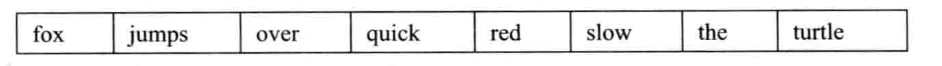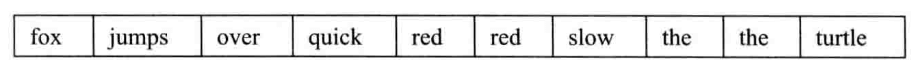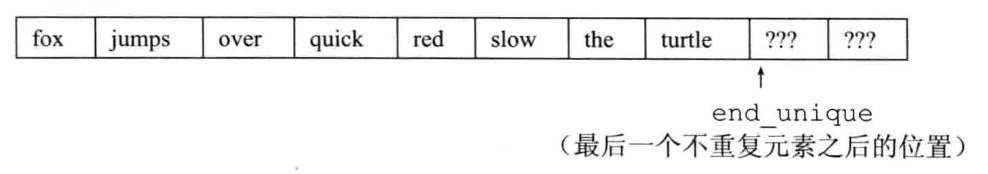# 【C++基础】第五十七课：[泛型算法]初识泛型算法

## accumulate，equal，fill，fill_n，back_inserter，copy，replace，replace_copy，sort，unique

Posted by x-jeff on November 27, 2022

【C++基础】系列博客为参考《C++ Primer中文版（第5版）》C++11标准）一书，自己所做的读书笔记。

# 2.只读算法

1
2
//对vec中的元素求和，和的初值是0
int sum = accumulate(vec.cbegin(), vec.cend(), 0);


accumulate的第三个参数的类型决定了函数中使用哪个加法运算符以及返回值的类型。

1
2
3
4
5
vector<int> vec = {1,2,3};
int sum = accumulate(vec.cbegin(),vec.cend(),0);
cout<< sum << endl; //输出6
sum = accumulate(vec.cbegin(),vec.cend(),2);
cout<< sum << endl; //输出8


## 2.1.算法和元素类型

accumulate将第三个参数作为求和起点，这蕴含着一个编程假定：将元素类型加到和的类型上的操作必须是可行的。即，序列中元素的类型必须与第三个参数匹配，或者能够转换为第三个参数的类型。在上例中，vec中的元素可以是int，或者是double、long long或任何其他可以加到int上的类型。

1
string sum = accumulate(v.cbegin(), v.cend(), string(""));


1
2
//错误：const char*上没有定义+运算符
string sum = accumulate(v.cbegin(), v.cend(), "");


## 2.2.操作两个序列的算法

1
2
//roster2中的元素数目应该至少与roster1一样多
equal(roster1.cbegin(), roster1.cend(), roster2.cbegin());


1
2
3
4
5
6
7
8
9
10
11
vector<int> vec1 = {1,2,3};
vector<int> vec2 = {1,2};
vector<int> vec3 = {1,2,3,4};
vector<float> vec4 = {1.f,2.f,3.f};
bool b;
b = equal(vec1.cbegin(),vec1.cend(),vec2.cbegin());
cout << b << endl; //打印0，即false，如果vec2的长度短于vec1，无论vec2里面是什么值，都返回0
b = equal(vec1.cbegin(),vec1.cend(),vec3.cbegin());
cout << b << endl; //打印1，即true，只要vec3和vec1中对应位置上的元素相等即返回1，不管vec3中多出的元素
b = equal(vec1.cbegin(),vec1.cend(),vec4.cbegin());
cout << b << endl; //打印1，即true


# 3.写容器元素的算法

1
2
3
fill(vec.begin(), vec.end(), 0); //将每个元素重置为0
//将容器的一个子序列设置为10
fill(vec.begin(), vec.begin() + vec.size()/2, 10);


## 3.1.算法不检查写操作

1
2
3
vector<int> vec;//空vector
//使用vec，赋予它不同值
fill_n(vec.begin(), vec.size(), 0);//将所有元素重置为0，vec其实依然是空的


1
fill_n(dest, n, val)


fill_n假定dest指向一个元素，而从dest开始的序列至少包含n个元素。

1
2
3
vector<int> vec;//空vector
//灾难：修改vec中的10个（不存在）元素，结果是未定义的
fill_n(vec.begin(), 10, 0);


## 3.2.介绍back_inserter

back_inserter接受一个指向容器的引用，返回一个与该容器绑定的插入迭代器。当我们通过此迭代器赋值时，赋值运算符会调用push_back将一个具有给定值的元素添加到容器中：

1
2
3
vector<int> vec = {1, 2, 3};
auto it = back_inserter(vec);//通过它赋值会将元素添加到vec中
*it = 42;//vec={1,2,3,42}


1
2
3
vector<int> vec;//空vector
//正确：back_inserter创建一个插入迭代器，可用来向vec添加元素
fill_n(back_inserter(vec), 10, 0);//添加10个元素到vec


## 3.3.拷贝算法

1
2
3
4
int a1[] = {0,1,2,3,4,5,6,7,8,9};
int a2[sizeof(a1)/sizeof(*a1)];//a2与a1大小一样
//ret指向拷贝到a2的尾元素之后的位置
auto ret = copy(begin(a1), end(a1), a2);//把a1的内容拷贝给a2，此时a1和a2完全一样


1
2
//将所有值为0的元素改为42
replace(ilst.begin(), ilst.end(), 0, 42);


1
2
3
4
list ilst = {0,1,0,1};
vector<int> ivec = {9,8,7};
//使用back_inserter按需要增长目标序列
replace_copy(ilst.cbegin(), ilst.cend(), back_inserter(ivec), 0, 42);//ivec={9,8,7,42,1,42,1}


# 4.重排容器元素的算法

1
the quick red fox jumps over the slow red turtle## 4.1.消除重复单词

1
2
3
4
5
6
7
8
9
10
void elimDups(vector<string>& words)
{
//按字典序排序words，以便查找重复单词
sort(words.begin(), words.end());
//unique重排输入范围，使得每个单词只出现一次
//排列在范围的前部，返回指向不重复区域之后一个位置的迭代器
auto end_unique = unique(words.begin(), words.end());
//使用向量操作erase删除重复单词
words.erase(end_unique, words.end());
}


sort算法接受两个迭代器，表示要排序的元素范围。在此例中，我们排序整个vector。完成sort后，words的顺序如下所示：## 4.2.使用unique

words排序完毕后，我们希望将每个单词都只保存一次。unique算法重排输入序列，将相邻的重复项“消除”，并返回一个指向不重复值范围末尾的迭代器。调用unique后，vector将变为：words的大小并未改变，它仍有10个元素。但这些元素的顺序被改变了—相邻的重复元素被“删除”了。我们将删除打引号是因为unique并不真的删除任何元素，它只是覆盖相邻的重复元素，使得不重复元素出现在序列开始部分。unique返回的迭代器指向最后一个不重复元素之后的位置。此位置之后的元素仍然存在，但我们不知道它们的值是什么。

## 4.3.使用容器操作删除元素